multiset

Sequence defined on multisets ★★

Author(s): Erickson

Conjecture   Define aarray of positive integers where the first row consists of some distinct positive integers arranged in increasing order, and the second row consists of any positive integers in any order. Create a new array where the first row consists of all the integers that occur in the first array, arranged in increasing order, and the second row consists of their multiplicities. Repeat the process. For example, starting with the array, the sequence is:->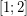->->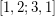->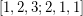->->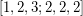->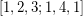->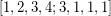->->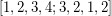->, and we now have a fixed point (loop of one array).

The process always results in a loop of 1, 2, or 3 arrays.

Keywords: multiset; sequence